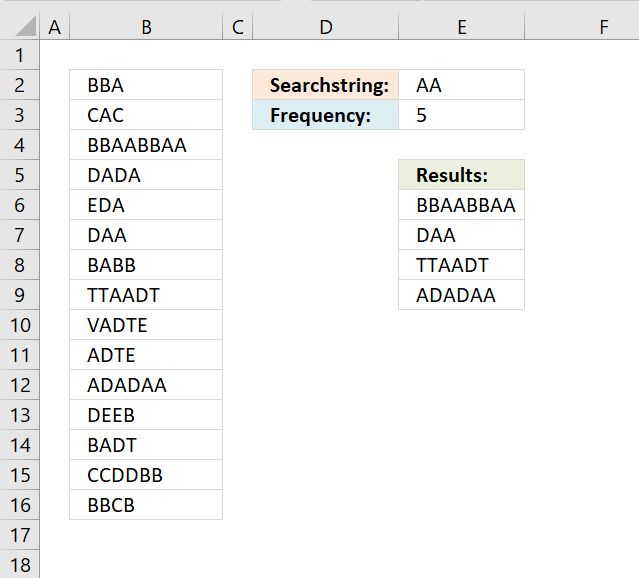Author: Oscar Cronquist Article last updated on November 17, 2018Question: How do I count how many times a word exists in a range of cells? It does not have to be an exact match but case sensitive. Column A1:A15 is the cell range.

Cell E2 is the search string. In cell E3 an array formula counts the number of times the search string is found in cell range A1:A15.

Case sensitive formula in cell E3:

=SUMPRODUCT((LEN(B2:B16)-LEN(SUBSTITUTE(\$B\$2:\$B\$16, \$E\$2, "")))/LEN(\$E\$2))

### Explaining formula in cell E3

#### Step 1 - Count characters in each cell

The LEN function counts characters in a cell.

LEN(B2:B16)

becomes

and returns

{3;3;8;4;3;3;4;6;5;4;6;4;4;6;4}

#### Step 2 - Substitue search string with nothing in all cells

The SUBSTITUTE function lets you replace a text string with another text string in a cell value or cell range.

SUBSTITUTE(\$B\$2:\$B\$16, \$E\$2, "")

becomes

and returns

#### Step 3 - Count characters in array

LEN(SUBSTITUTE(\$B\$2:\$B\$16, \$E\$2, ""))

becomes

and returns

{3;3;4;4;3;1;4;4;5;4;4;4;4;6;4}

#### Step 4 - Subtract arrays

LEN(B2:B16)-LEN(SUBSTITUTE(\$B\$2:\$B\$16, \$E\$2, ""))

becomes

{3;3;8;4;3;3;4;6;5;4;6;4;4;6;4}-{3;3;4;4;3;1;4;4;5;4;4;4;4;6;4}

and returns

{0;0;4;0;0;2;0;2;0;0;2;0;0;0;0}

#### Step 5 - Divide with cell length

(LEN(B2:B16)-LEN(SUBSTITUTE(\$B\$2:\$B\$16, \$E\$2, "")))/LEN(\$E\$2

becomes

{0;0;4;0;0;2;0;2;0;0;2;0;0;0;0}/LEN(\$E\$2)

becomes

{0;0;4;0;0;2;0;2;0;0;2;0;0;0;0}/{3;3;8;4;3;3;4;6;5;4;6;4;4;6;4}

and returns

{0;0;0.5;0;0;0.666666666666667;0;0.333333333333333;0;0;0.333333333333333;0;0;0;0}

#### Step 6 - Sum number sin array

The SUMPRODUCT is better in this case because you are not required to enter the formula as an array formula to do the calculations.

SUMPRODUCT((LEN(B2:B16)-LEN(SUBSTITUTE(\$B\$2:\$B\$16, \$E\$2, "")))/LEN(\$E\$2))

becomes

SUMPRODUCT({0;0;0.5;0;0;0.666666666666667;0;0.333333333333333;0;0;0.333333333333333;0;0;0;0})

and returns 5 in cell E2.

Case insensitive formula in cell E3:

=SUMPRODUCT((LEN(B2:B16)-LEN(SUBSTITUTE(UPPER(\$B\$2:\$B\$16), UPPER(\$E\$2), "")))/LEN(\$E\$2))

Array formula in cell E6:

=IFERROR(INDEX(\$A\$1:\$A\$15, SMALL(IF(ISNUMBER(FIND(\$D\$1, \$A\$1:\$A\$15)),  MATCH(ROW(A1:A15), ROW(A1:A15)), ""), ROW(A1))), "")